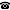# 2008 seminar talk: Strong reflection

Talk held by James Cummings (Carnegie Mellon University) at the KGRC seminar on 2008-06-19.

### Abstract

(joint work with Dorshka Wylie) Let $S, T$ be stationary subsets of an uncountable regular $\kappa$. Say that $S <^* T$ iff every stationary subset of $S$ reflects at almost every point in $T$. Let $S^n_j = \{ \alpha < \omega_n : cf(\alpha) = \omega_j \}$. We construct a model with a sequence of stationary sets $B_n \subseteq S^{n+2}_{n+1}$ for $n < \omega$, such that $S^{n+2}_i <^* B_n$ for all $i \le n$, in which the sets $B_n$ are as large as possible (it is not possible that $B_n = S^{n+2}_{n+1}$).

Kurt Gödel Research Center for Mathematical Logic. Währinger Straße 25, 1090 Wien, Austria.+43-1-4277-50501. Last updated: 2010-12-16, 04:37.